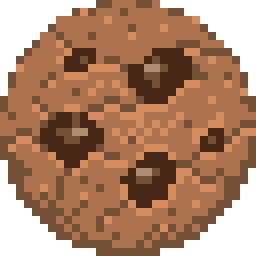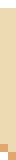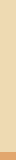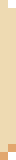# Math 1011. Which of the following numbers are prime?
A21 B31 C32 D45 E50
2. Which of the following numbers can be written as the sum of the side lengths of a right triangle with sides of integer length?
A1 B4 C12 D14 E60
3. Which of the following numbers can be written as the sum of three squares?
A27 B100 C215 D247 E700
4. Which of the following are a number of degrees whose cosine is positive?
A210 B270 C455 D620 E650
5. Which of the following numbers are perfect squares?
A2 B3 C4 D5 E6
6. Which of the following numbers are abundant?
A4 B19 C20 D22 E24
7. Which of the following numbers can be written as the sum of two consecutive integers?
A0 B2 C4 D5 E7
8. Which of the following numbers are palindromes in octal?
A95 B121 C261 D279 E471
9. Which of the following numbers are a leap year?
A2014 B2020 C2628 D2911 E3200
10. Which of the following numbers appear at least three times in Pascal’s triangle?
A2 B22 C58 D92 E126
11. Which of the following numbers can be written as the sum of two primes?
A23 B30 C39 D47 E59